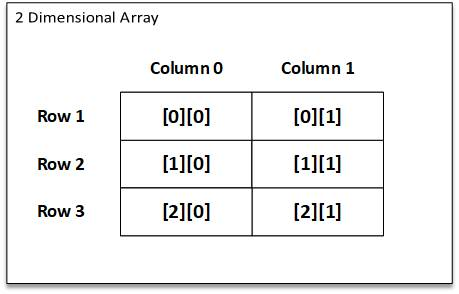# Array C Programing

C allows us to define tables (collection of row and columns) by using two dimensional arrays. Two dimensional arrays are declared as follows:

type array-name [row-size][column-size];

For Example:

``````
int number;

``````

will create a table of 3 rows and 2 columns. Its representation in memory looks like below. The first index represent row and the second index selects column in that row.Representation of two dimesional array in memory.

Initialization of two dimensional memory:

The two dimensional arrays may be initialized by following their declaration with the list of initial values enclosed in braces.

For Example:

``````
int n={000,111};

``````

The above will initialize the first row to zero and second row to one. The initialization is done row by row. The above statement can also be written as:

``````
int n={{0,0,0},{1,1,1}}

``````

by surrounding the elements of each row by brces.

When the array is completely initialized with all values, explicitly, we not need to  specify the size of the first dimension.

For Example:

``````
int n[]={{0,0,0},{1,1,1}}

``````

If the values are missing in an intializer, they are automatically set to zero.

For Example:

``````
int={{1,1},{2}};

``````

will initialize the first two elements of the first row to one, the first element of second row to two and all other elements to zero.